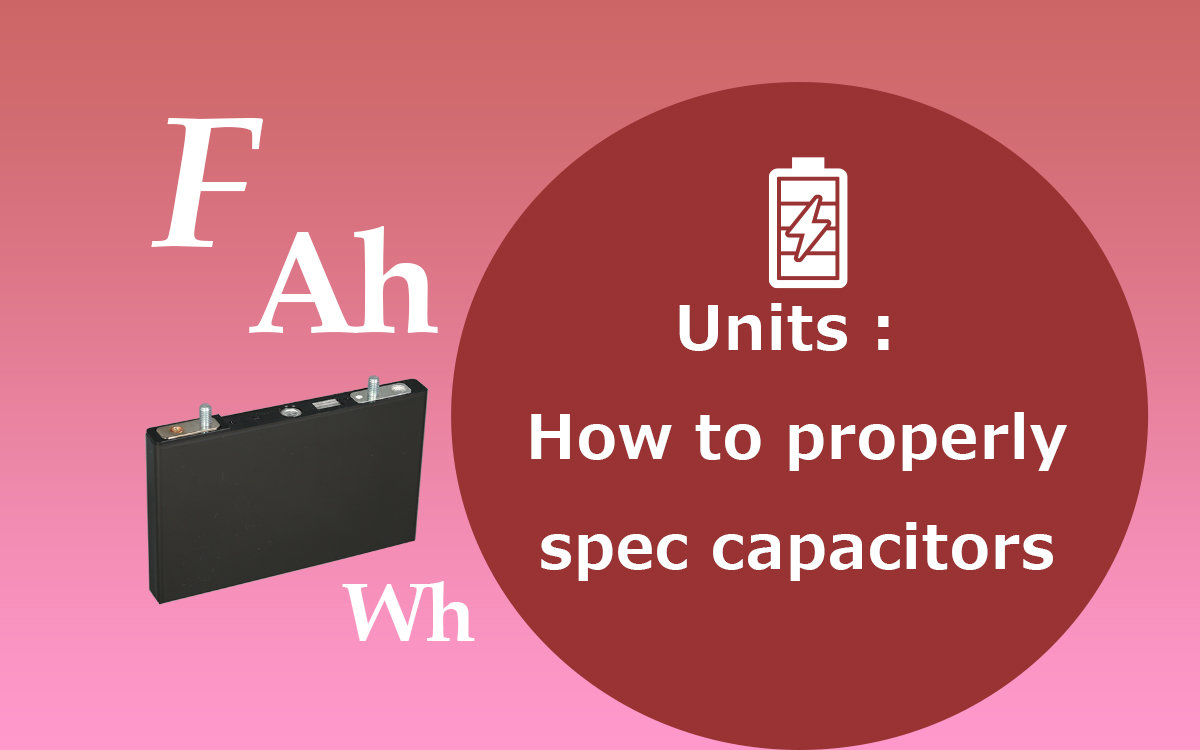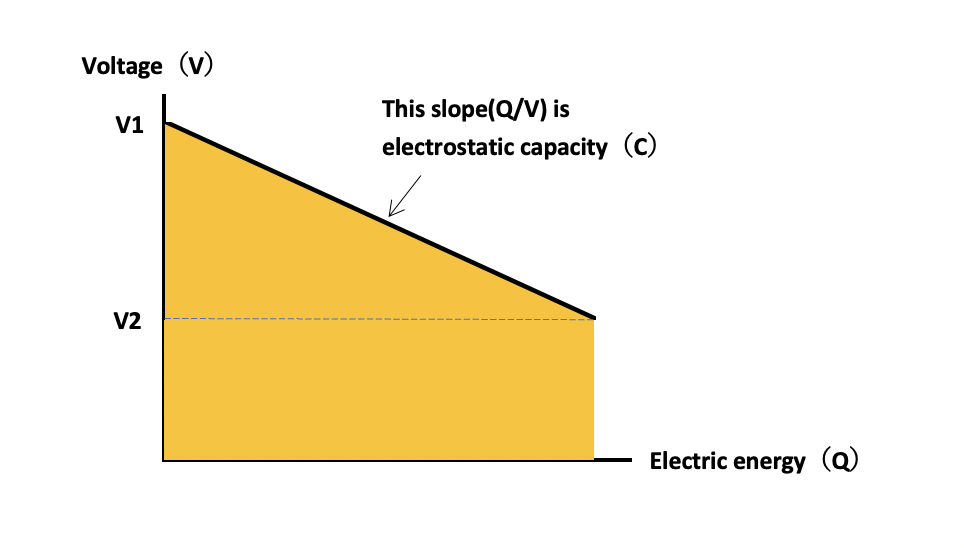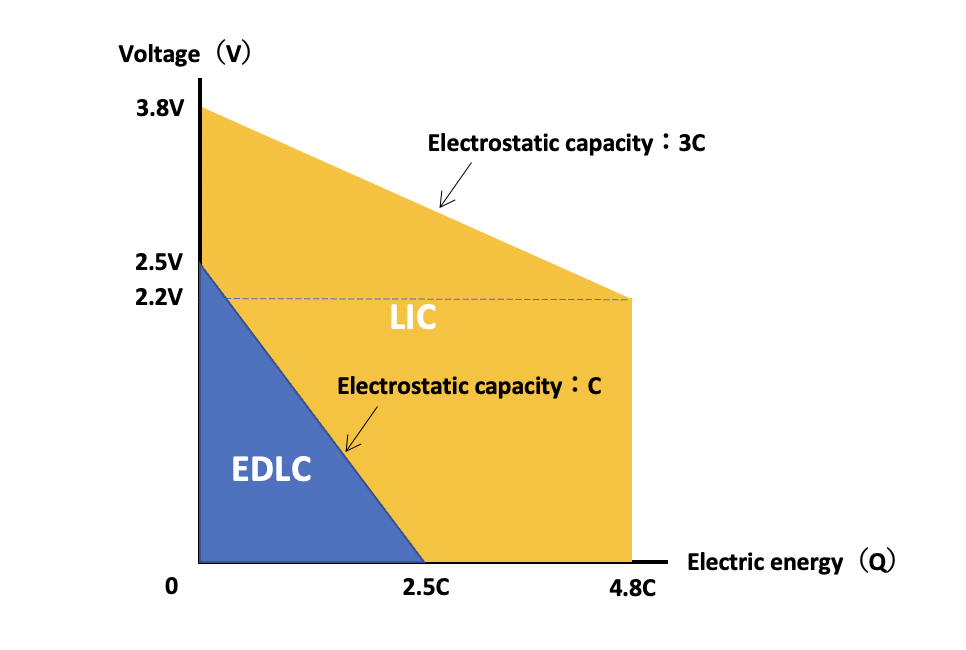# Blog

Read blog articles of Musashi Energy Solutions Co.，Ltd..

# Relationship between capacitor voltage and energy contentF (farad), Ah (amp-hour), Wh (watt-hour), etc. are often used as units that express the characteristics of capacitors, but many people may not understand the contents. This time, I will explain the meaning of these units and their relationships.

If you have a capacitor, you will definitely see the indications such as F and μF.

This is called capacitance, which is a quantity that expresses how much charge can be stored in the capacitor. Even with the same power storage device, many people may be confused because it is a unit that cannot be seen with batteries. In fact, the essential difference between capacitors and batteries is hidden here.

As explained in "What is a capacitor?", the difference between a capacitor and a battery lies in how electricity is stored. Unlike batteries, which store electricity through chemical reactions, capacitors store electricity on the surface of the electrodes by the force of static electricity, so the voltage (V) changes linearly in proportion to the stored electricity (Q = CV).

C at this time is called capacitance, and corresponds to the slope when the relationship between the amount of electricity and voltage is represented by a graph.## Relationship between F, Ah and Wh

The amount of electricity (Q) on the horizontal axis is the amount of electric charge stored in the capacitor and is expressed in units such as Ah. The amount of electricity that can be stored or consumed in one hour with a current of 1A is 1Ah.

In the world of batteries, the voltage is constant even if electricity is consumed, so the amount of energy that can be stored is expressed by multiplying the voltage and the amount of electricity. The unit of this amount of energy is V ・ Ah ＝ Wh.

Since the voltage of one capacitor changes linearly, the average voltage (V1 + V2) / 2 during this period is used to calculate the amount of energy. That is, the amount of energy is (V1 + V2) x Q / 2.

Have you noticed anything by looking at this formula and graph? that's right. The amount of energy is nothing but the area of ​​a trapezoid surrounded by the amount of voltage and electricity.

When I saw the units such as F, Ah, and Wh of the capacitor, I wanted to be able to imagine this trapezoid in my head. It will be a powerful tool to help you understand capacitors, such as the difference between capacity (electricity) and capacitance: capacity, which are often confused, and the amount of energy that works with the square of the voltage.

## Amount of energy of lithium ion capacitor

A lithium ion capacitor (LIC) uses a carbon-based material that can store lithium ions as a negative electrode material while using the principle of a general electric double layer capacitor (EDLC) (see this blog for EDLC). It is a capacitor with improved energy density by adding lithium ions to it.

Let's compare the amount of energy of this lithium ion capacitor and electric double layer capacitor using the graph explained earlier.Let's assume that both sizes are the same and that the same activated carbon is used for the positive electrode. Unlike electric double layer capacitors that use activated carbon for the negative electrode, the negative electrode of lithium ion capacitors has a much larger capacity, so the capacitance as a cell is about 3 compared to electric double layer capacitors of the same size. Double is expected.

• Since the electric quantity Q = CV of the electric double layer capacitor (operating voltage: 0 to 2.5V) with capacitance C is 2.5C, the energy quantity is (V1 + V2) x Q / 2 = 2.5 x 2.5C / 2 = 3.1C.
• On the other hand, the amount of electricity Q of a lithium-ion capacitor (operating voltage: 2.2 to 3.8V) with a capacitance of 3C is 3C x (3.8-2.2) = 4.8C, and the amount of energy is (3.8 + 2.2) x 4.8C / 2. = 14.4C.

In other words, it can be seen that the lithium ion capacitor has 14.4 / 3.1 = 4.6 times more energy than the electric double layer capacitor (EDLC) of the same size.

## Summary

How was that?

This time, we introduced the units related to capacitors.

We are developing and manufacturing the lithium-ion capacitors introduced today. If you are interested, please refer to it.

* Please understand that the description in this blog includes interpretations based on the author's experience and subjective opinions. In addition, the technical information contained in this blog does not grant any guarantee or license to the intellectual property rights or other rights of the Company or a third party.

Blog Category
Annual Archive